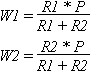# DIY Audio & Video

## Inductors, Capacitors, and Resistors

Simply put inductors, capacitors, and resistors have inductance, capacitance, and resistance. The problem is that all three electronic components have all three properties. When designing a circuit for a speaker system, it is assumed that inductors have only inductance, capacitors have capacitance, and resistors have resistance. This is not true, and can cause major problems when designing circuits.

For an example, look at a 1st order low pass Butterworth crossover at 100 Hz for an 8 ohm speaker. The formula calls for a 12.75 mH inductor in series with the driver. This value is based on the resistance of the driver and the crossover point. A 12.75 mH inductor made from 18 gauge wire will have a resistance above 1.5 Ohms. This resistance is caused by the resistance of the copper wire. This makes the resistance of the driver and circuit 9.5 Ohms. This change in resistance will cause the 12.75 mH inductor to become a low pass crossover at 118 Hz instead of 100 Hz. Also, because the resistance of the driver and circuit has increased, the amplifier will put out less power and this driver will not play as loud.

This is just one example of what can happen. There are many others. There are also several solutions. The simple solution is to accept it and forget it. Most of the time, the effect will not be as bad as in the above example. It takes a low crossover point to require a large inductor.

The second option is to factor this effect into your equations. A 15.6 mH inductor has a resistance of approximately 1.8 Ohms. This is normally a 80 Hz low pass crossover for a 8 Ohm driver, but for a 9.8 Ohm driver, it is a 100 Hz low pass crossover. It will take several attempts to get the numbers to work out, but it is possible. Remember that this does not help the problem with the amplifier seeing a greater load. It actually made the problem worse.

The third option is to account for the effect in another component. A series notch filter uses a capacitor, inductor, and resistor. If the inductor has a resistance of 1 Ohm, then simply use a 1 Ohm smaller resistor than what is called for. Refer to the Basic Electronics for information on how multiple resistors work in the same circuit.

The final option is to use better quality electronic components, so that the effect is minimized. For inductors, this is simple. Use a heavier gauge wire to lower the resistance of the wire. You can wind your own inductors with any gauge wire you want. Changing the coil size can also have a small effect on the amount of wire needed, and the resistance. Iron core inductors use much less wire, but at the cost of performance. They heat up easier, and then their inductance will begin to change. For capacitors, there are several types that can be purchased. The basic electrolytic capacitors are cheap, but do not pass high frequencies well. Mylar capacitors pass high frequencies better, and have less inductance and resistance. Polypropylene are even more expensive, but have the least problems. It is suggested that you use Mylar or Polypropylene capacitors for values less than 20 uF in the mid or tweeter section of the circuit. Otherwise, use a non-polar electrolytic capacitor.

Resistors also have a wattage rating that determines how much power they can handle. As a general rule, any resistor in series or in parallel with the driver needs to be able to handle as many watts as the driver will see. A resistor in line with a 100W driver needs to be rated at 100W. The only special case is resistors used in a L-pad. For a L-Pad the two resistors should sum to the wattage of the driver.

W1 = Wattage rating of 1st resistor in Watts

W2 = Wattage rating of 2nd resistor in Watts

R1 = Resistance of 1st resistor in Ohms

R2 = Resistance of 2nd resistor in Ohms

P = Power that the driver can handle in WattsExample: A 6 dB pad for an 8-ohm driver driven by a 100 watt source calls for an 8 and a 4 ohm resistor. The required power rating of each resistor is 100*8/(8+4) = 66.6W and 100*4/(8+4)=33.3W Both of the resistor wattage ratings add up to 100.